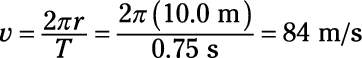##### Physics I Workbook For Dummies with Online Practice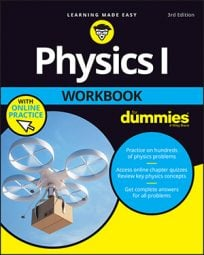When describing the way things go in circles, you don’t just use radians; you also can specify the time it takes. The time it takes for an object to complete an orbit is referred to as the period of its motion. Period is generally measured in seconds, but it can be measured using other units of time, including milliseconds, minutes, and years.

If an object is traveling at speed v, then the time it takes to go around the circle — the distance it travels in the circle’s circumference, 2π r — will be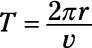Note the symbol of the radius of a circle: r. That’s half the circle’s diameter, which is d. So r = d/2. Note also the symbol for the period: T. With this equation, given an orbiting object’s speed and the radius of the circle, you can calculate the object’s period.

Another measurement you’ll see in physics problems is frequency. Whereas the period is the time an object takes to go around in a circle, the frequency is the number of circles the object makes per unit of time. The frequency, f, is connected to the period like this:

Frequency is most often measured in units of cycles per second (cps), which are also called Hertz (Hz). An object moving in a circle with a frequency of 2.0 Hz completes two trips around its circle every second.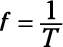## Sample question

1. The moon’s orbital radius is 3.85 x 108 m, and its period is about 27.3 days. What is its speed as it goes around Earth?

The correct answer is 1,020 m/s, when rounded for significant figures.

1. Convert 27.3 days to seconds: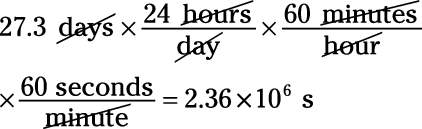2. Use the equation for the period to solve for speed: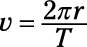3. Plug in the numbers: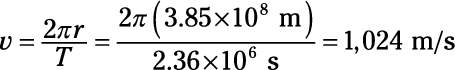## Practice questions

1. You have a ball on a string, and you’re whipping it around in a circle. If the radius of its circle is 1.0 m and its period is 1.0 s, what is its speed?

2. You have a toy plane on a wire, and it’s traveling around in a circle. If the radius of its circle is 10.0 m and its period is 0.75 s, what is its speed?

Following are answers to the practice questions:

1. 6.3 m/s

1. Use the equation for the period to solve for speed: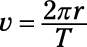2. Plug in the numbers: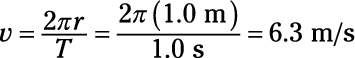2. 84 m/s

1. Use the equation for the period to solve for speed:2. Plug in the numbers: